# NCERT Solutions for Class 10 Maths Chapter 12 Areas Related to Circles Ex 12.3

NCERT Solutions for Class 10 Maths Chapter 12 Areas Related to Circles Ex 12.3 are part of NCERT Solutions for Class 10 Maths. Here are we have given Chapter 12 Areas Related to Circles Class 10 NCERT Solutions Ex 12.3.

 Board CBSE Textbook NCERT Class Class 10 Subject Maths Chapter Chapter 12 Chapter Name Areas Related to Circles Exercise Ex 12.3 Number of Questions Solved 16 Category NCERT Solutions

## NCERT Solutions for Class 10 Maths Chapter 12 Areas Related to Circles Ex 12.3

Page No: 234

Unless stated otherwise, use π =22/7

Question 1.
Find the area of the shaded region in Fig. 12.19, if PQ = 24 cm, PR = 7 cm and O is the centre of the circle.

Solution: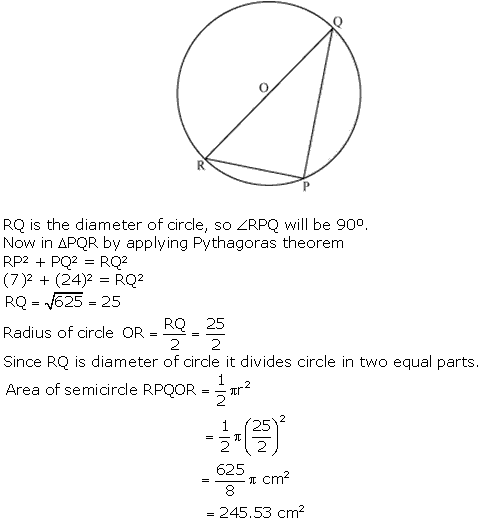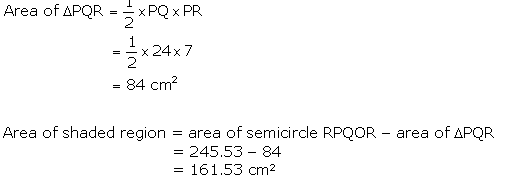Question 2.
Find the area of the shaded region in Fig. 12.20, if radii of the two concentric circles with centre O are 7 cm and 14 cm respectively and ∠AOC = 40°.

Solution: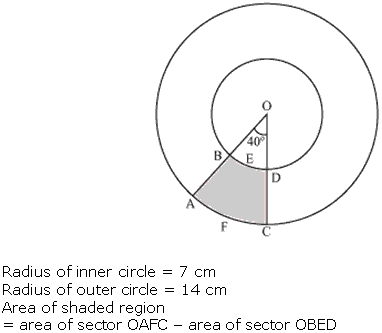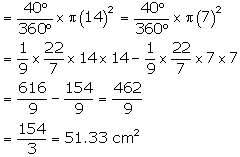Question 3.
Find the area of the shaded region in Fig. 12.21, if ABCD is a square of side 14 cm and APD and BPC are semicircles.

Solution: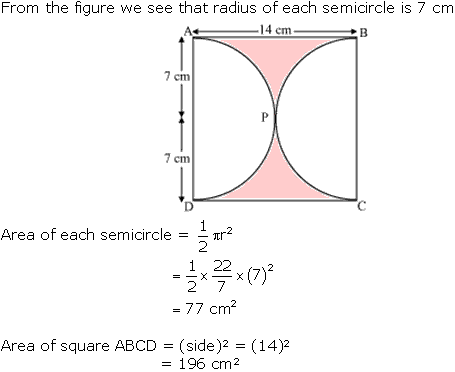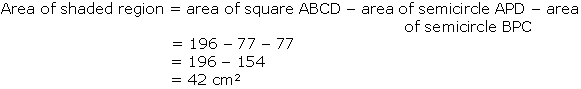Question 4.
Find the area of the shaded region in Fig. 12.22, where a circular arc of radius 6 cm has been drawn with vertex O of an equilateral triangle OAB of side 12 cm as centre.

Solution: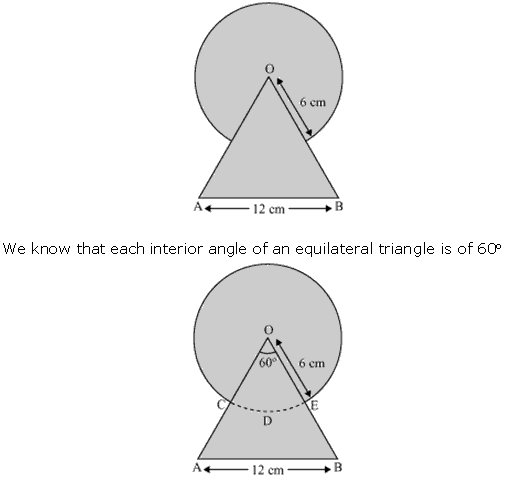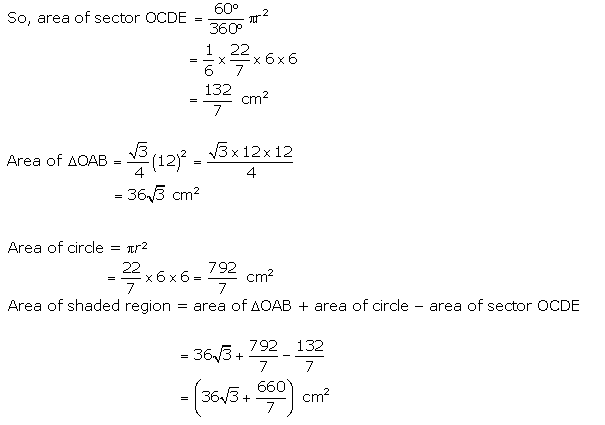Question 5.
From each corner of a square of side 4 cm a quadrant of a circle of radius 1 cm is cut and also a circle of diameter 2 cm is cut as shown in Fig. 12.23. Find the area of the remaining portion of the square.

Solution: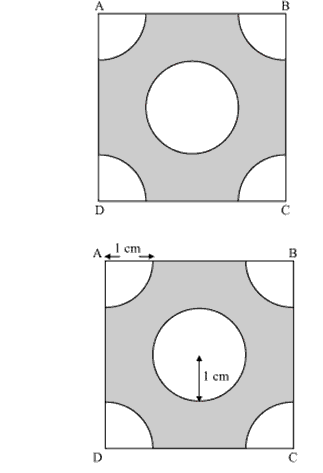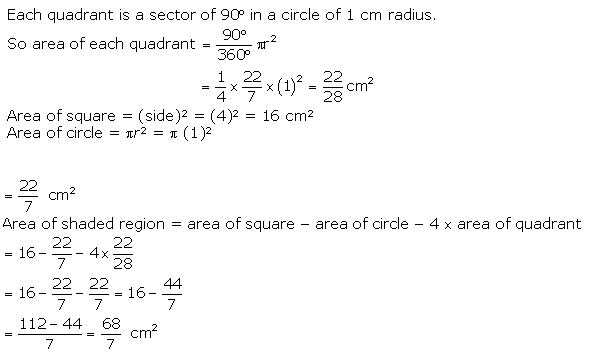Question 6.
In a circular table cover of radius 32 cm, a design is formed leaving an equilateral triangle ABC in the middle as shown in Fig. 12.24. Find the area of the design.

Solution: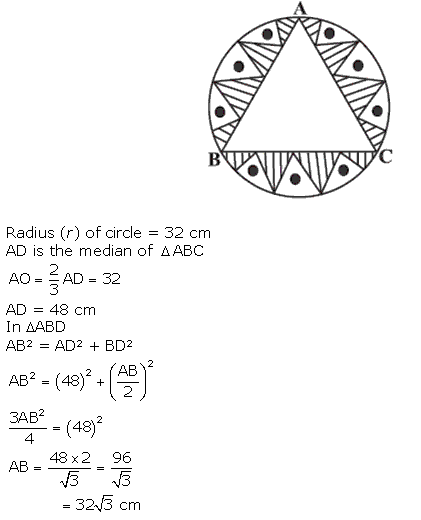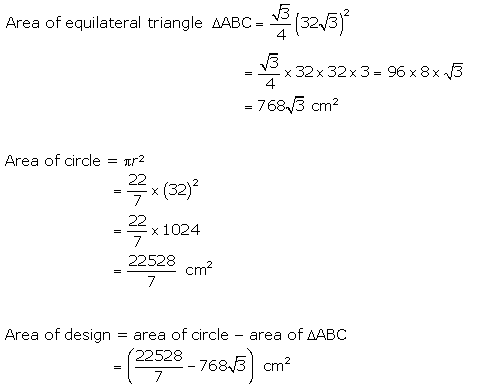Page No: 236

Question 7.
In Fig. 12.25, ABCD is a square of side 14 cm. With centres A, B, C and D, four circles are drawn such that each circle touch externally two of the remaining three circles. Find the area of the shaded region.

Solution: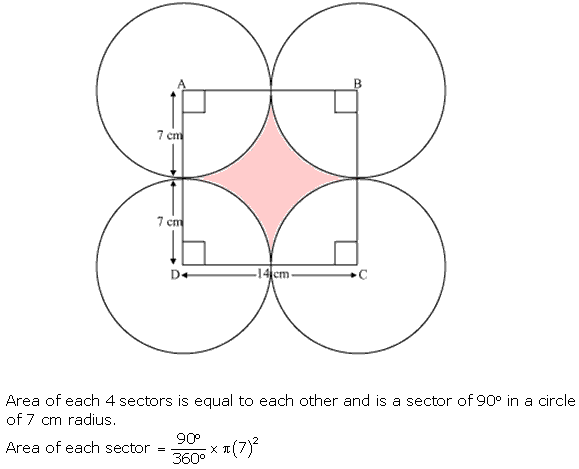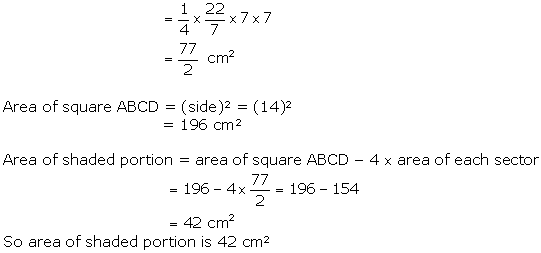Question 8.
Fig. 12.26 depicts a racing track whose left and right ends are semicircular.
The distance between the two inner parallel line segments is 60 m and they are each 106 m long. If
the track is 10 m wide, find :
(i) the distance around the track along its inner edge
(ii) the area of the track.

Solution: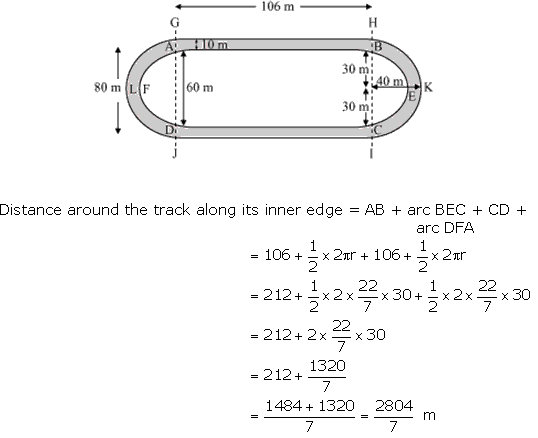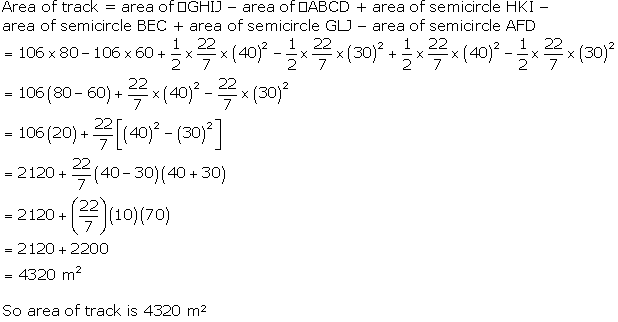Question 9.
In Fig. 12.27, AB and CD are two diameters of a circle (with centre O) perpendicular to each other
and OD is the diameter of the smaller circle. If OA = 7 cm, find the area of the shaded region.

Solution: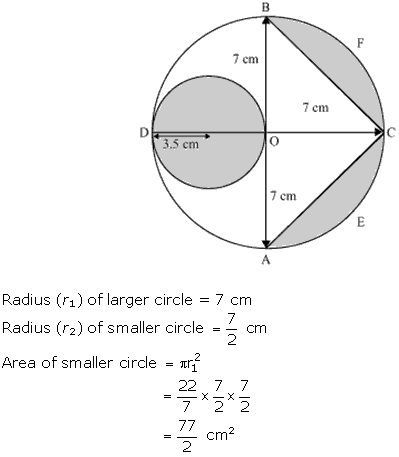Question 10.
The area of an equilateral triangle ABC is 17320.5 cm2. With each vertex of the triangle as centre, a circle is drawn with radius equal to half the length of the side of the triangle (see Fig. 12.28). Find the area of the shaded region. (Use π = 3.14 and √3 = 1.73205)

Solution: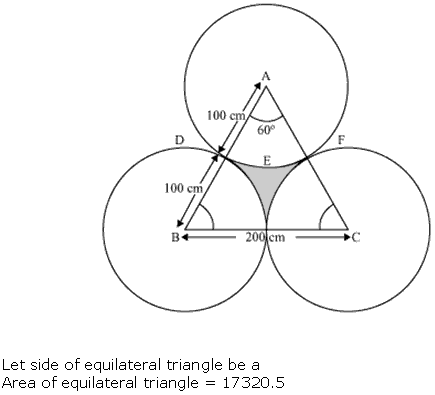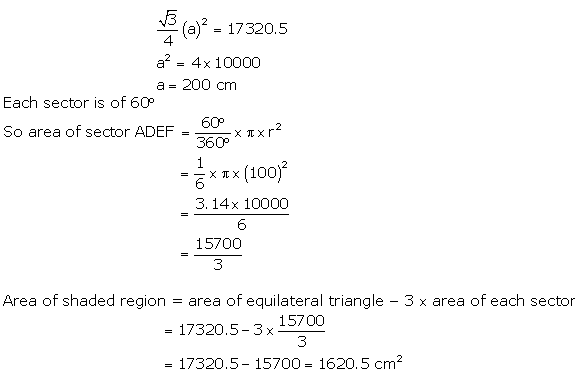Question 11.
On a square handkerchief, nine circular designs each of radius 7 cm are made (see Fig. 12.29). Find the area of the remaining portion of the handkerchief.

Solution: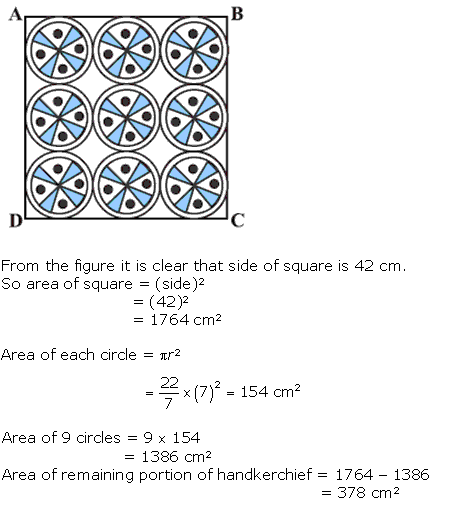Question 12.
In Fig. 12.30, OACB is a quadrant of a circle with centre O and radius 3.5 cm. If OD = 2 cm, find the area of the

Solution: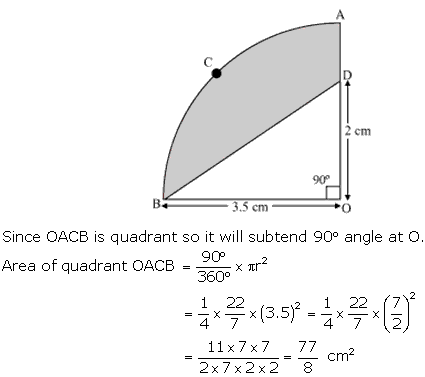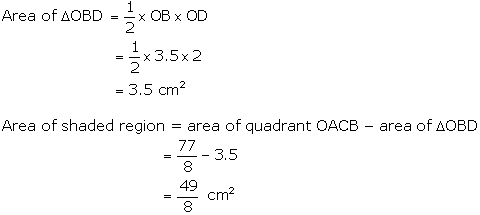Question 13.
In Fig. 12.31, a square OABC is inscribed in a quadrant OPBQ. If OA = 20 cm, find the area of the shaded region. (Use π = 3.14)

Solution: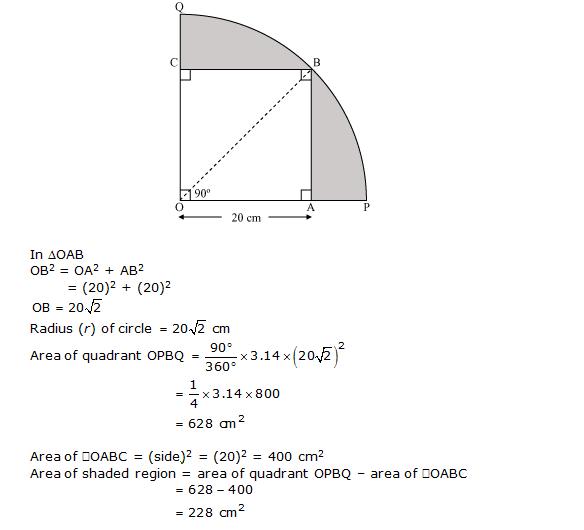Question 14.
AB and CD are respectively arcs of two concentric circles of radii 21 cm and 7 cm and centre O (see Fig. 12.32). If ∠AOB = 30°, find the area of the shaded region.

Solution: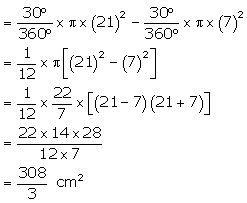Question 15.
In Fig. 12.33, ABC is a quadrant of a circle of radius 14 cm and a semicircle is drawn with BC
as diameter. Find the area of the shaded region.

Solution: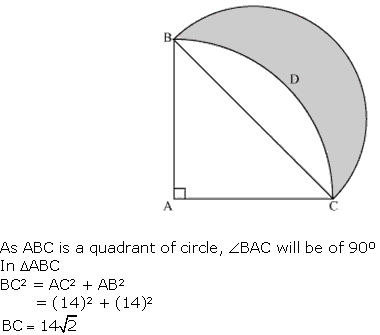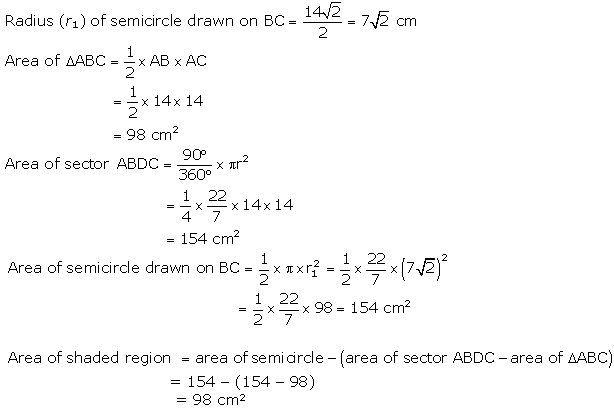Page No: 238

Question 16.
Calculate the area of the designed region in Fig. 12.34 common between the two quadrants of circles of radius 8 cm each.

Solution: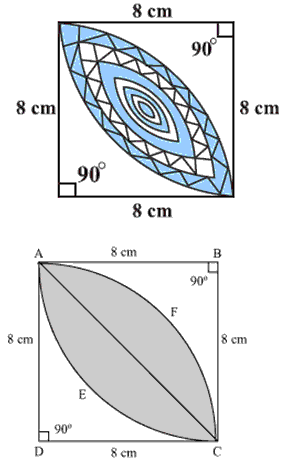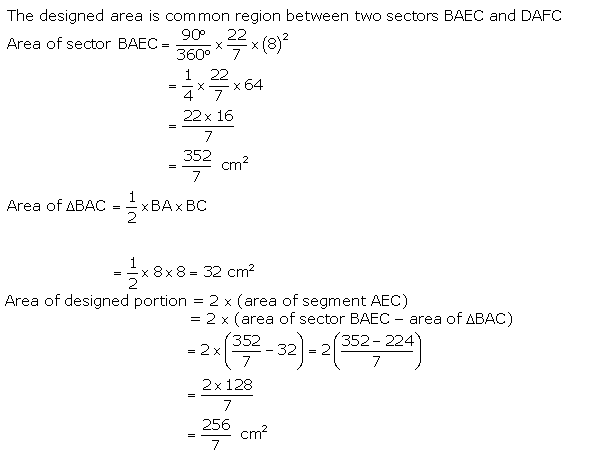We hope the NCERT Solutions for Class 10 Maths Chapter 12 Areas Related to Circles Ex 12.3 help you. If you have any query regarding NCERT Solutions for Class 10 Maths Chapter 12 Areas Related to Circles Ex 12.3, drop a comment below and we will get back to you at the earliest.